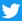## Derek Jeter's 3000th Hit (7/9/11)## Kinematic Data:

initial
position (ft)
initial
velocity (ft/s)
average
acceleration (ft/s2)
xo = 2.155 vxo = -4.427 ax = -2.697
yo = 50.00 vyo = -114.794 ay = 18.983
zo = 6.191 vzo = -1.747 az = -33.818

Initial Speed (mph): 78.3
Speed at the front of home plate (mph): 73.6
Position at the front of home plate (ft):
x = -0.049, y = 1.417, and z = 2.162

Maintained by Dr. Baseball, Ph.D. ()